﻿ 柱坐标在绞吸式挖泥船绞刀齿座安装中的应用 Application of Column Coordinates in the Installation of the Cutter of the Cutter Suction Dredger

Mechanical Engineering and Technology
Vol. 08  No. 05 ( 2019 ), Article ID: 32576 , 8 pages
10.12677/MET.2019.85047

Application of Column Coordinates in the Installation of the Cutter of the Cutter Suction Dredger

Yong Zhang1, Yuan Ma1, Chun-Tian Sun2

1CCCC National Engineering Research Center of Dredging Technology and Equipment Co. Ltd., Shanghai

2Shanghai Waigaoqiao Ship Building Co. Ltd., Shanghai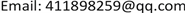Received: Sep. 27th, 2019; accepted: Oct. 11th, 2019; published: Oct. 18th, 2019ABSTRACT

Since the construction efficiency of the cutter suction dredger is closely related to the performance of the cutter head, in order to improve the construction efficiency of the cutter suction dredger, this paper refers to the cylindrical coordinate method to locate the cutter holder and the teeth. By designing and manufacturing a simple device, and selecting three positioning points on the adapter, the mounting position of the cutter holder is determined according to the cylindrical coordinates of the three positioning points of the adapter. In the actual production and construction, the problem of installation and positioning of the reamer teeth and the tooth holder is effectively solved.

Keywords:Column Coordinates, Positioning Work, Cutter Suction Dredger, Adapters

1中交疏浚技术装备国家工程研究中心有限公司，上海

2上海外高桥造船有限公司，上海1. 引言

2. 齿座安装位置确定

2.1. 确定刀齿安装线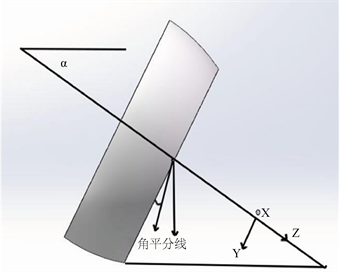Figure 1. Tool mounting line coordinate system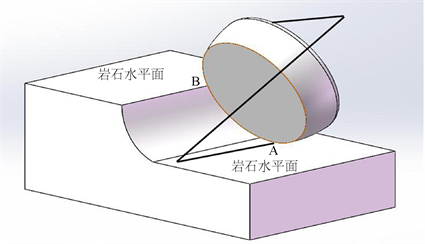Figure 2. Reamer construction simulation

2.2. 刀齿安装位置的确定

$\left\{\begin{array}{l}x=\frac{d}{2}\mathrm{sin}\left(\Omega \right)\mathrm{sin}\left(\theta \right)\hfill \\ y=\frac{\left(N-1\right)D}{2N}\mathrm{cos}\left(\theta \right)+kH\mathrm{sin}\left(\theta \right)+\frac{D}{2N}\hfill \end{array}$ (1)

$\left\{\begin{array}{l}x=\frac{d}{2}\mathrm{sin}\left(\Omega \right)\mathrm{sin}\left(\theta \right)-B\mathrm{sin}\left(\varphi \right)\hfill \\ y=\left[\frac{\left(N-1\right)D}{2N}-B\mathrm{cos}\left(\theta \right)\right]\mathrm{cos}\left(\theta \right)+kH\mathrm{sin}\left(\theta \right)+\frac{D}{2N}\hfill \end{array}$ (2)

$\left\{\begin{array}{l}x=\frac{d}{2}\mathrm{sin}\left(\Omega \right)\mathrm{sin}\left(\theta \right)\hfill \\ y=\frac{\left(N-1\right)D}{2N}-+kH\mathrm{sin}\left(\theta \right)+\frac{D}{2N}\hfill \\ z=H\mathrm{sin}\left(\theta \right)-\frac{k\left(N-1\right)D}{2N}\mathrm{cos}\left(\theta \right)+k\frac{\left(N-1\right)D}{2N}\hfill \end{array}$ (3)

3. 绞刀齿、齿座的设计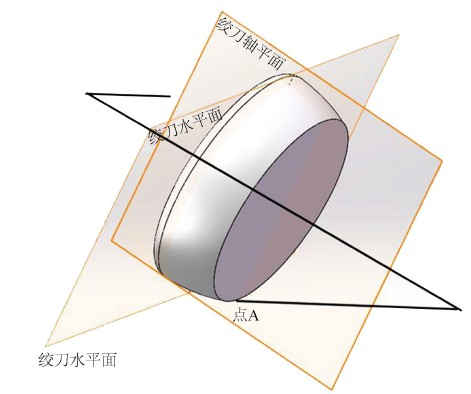Figure 3. Reamer projection surface

$\mathrm{tan}{\theta }_{XOY}=\mathrm{cos}\beta \mathrm{tan}\frac{\alpha }{2}$$\mathrm{tan}{\theta }_{YOZ}=\mathrm{cos}\frac{\alpha }{2}\mathrm{tan}\beta$$\mathrm{tan}{\theta }_{XOZ}=\left[\mathrm{tan}2\left(\frac{\alpha }{2}\right)+\mathrm{tan}2\beta \right]-\frac{1}{2}$ (1)

4. 绞刀头座的柱坐标表达Figure 4. Column coordinate representation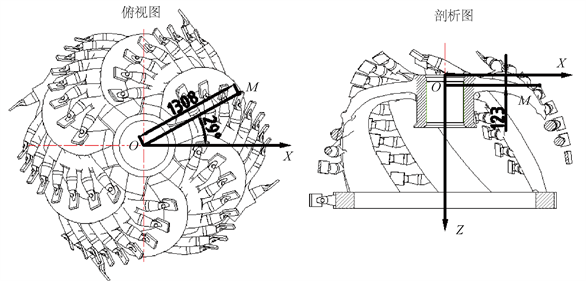Figure 5. The expression of cylindrical coordinates in the reamer model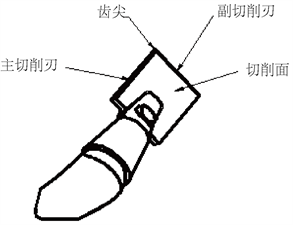Figure 6. Wrench cutter diagram

5. 利用柱坐标法设计制作简易工装实现绞刀齿座定位Figure 7. Schematic diagram of the reaper tooth holder

6. 总结

Application of Column Coordinates in the Installation of the Cutter of the Cutter Suction Dredger[J]. 机械工程与技术, 2019, 08(05): 408-415. https://doi.org/10.12677/MET.2019.85047

1. 1. 安传志. 改善绞吸挖泥船绞刀油马达工作能力分析[J]. 中国设备工程, 2016(10):42-43.

2. 2. 曹红亮. 绞吸式挖泥船液压系统故障分析以及维护[J]. 山东工业技术, 2015(5): 46-46.

3. 3. 金晔. 大型绞吸挖泥船设计关键技术分析[J]. 建筑技术开发, 2017, 44(16): 73-75.

4. 4. 孟昭生, 王家印, 陈宗源. 绞吸挖泥船操作人员技术手册[M]. 北京: 中国交通建设集团, 2006.

5. 5. 杨桢毅. 绞吸式挖泥船绞刀结构与性能优化的研究[D]: [硕士学位论文]. 武汉: 武汉理工大学, 2010.

6. 6. 杨啸轶, 杨启, 刘丹. 绞吸式挖泥船绞刀臂轮廓线和刀齿安装角的确定方法[J]. 港工技术, 2013, 50(4): 31-34.

7. 7. 同济大学数学系. 高等数学第六版下册[M]. 上海: 高等教育出版社, 2007.

8. 8. 尤宝, 梁晓辉. 泵体水力模具逆向工程技术研究[J]. 制造业自动化, 2018, 40(9): 138-142+150.